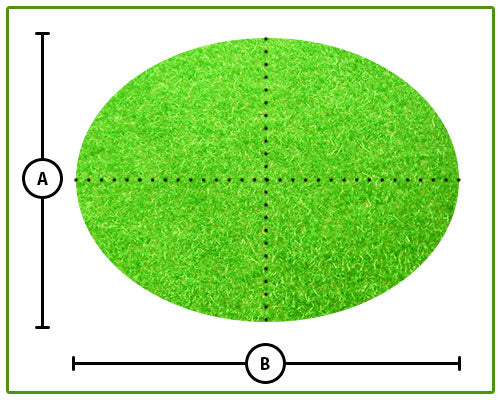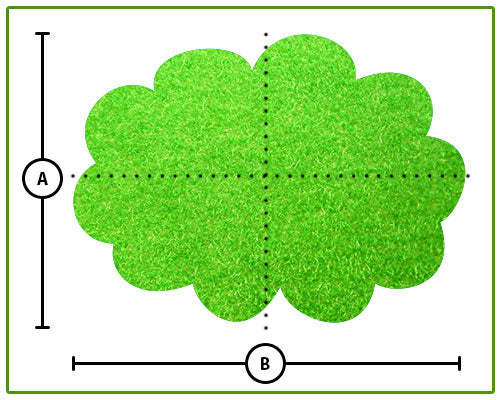##### 0845 600 8633

8pm - 5pm (Mon-Fri)

# How to Measure for Artificial Grass

If you are looking at buying artificial grass then you need to make sure you are ordering enough to completely cover your lawn or chosen installation space before you commit to the purchase.

To help make things easier for you take a look through this section of our website to find out exactly how best to measure square and “uneven” square areas such as lawns and patios, round or circular areas such as grass centerpieces and features, “L” shaped areas such as a lawn that wraps around 2 sides of a house and other shapes that at first seem difficult to measure such as triangles and other “uneven” shapes that are common for gardens.

Direct Artificial Grass provide many of our products in 2M & 4M lengths to help make buying the right amount of artificial grass easier for you.

### How To Measure A Square or Rectangular Garden or AreaTo measure a square area or garden is very easy. All you need to do is multiply the longest length by the longest width in metres to get the total area in square meters(sqm).

Look at the picture to the right, the length is “A” and the width is “B“.

So, if the length (A) is 4m and the width (B) is 4m then your total area is;

(A)4m x (B)4m = 16/sqm total

### How to Measure an Uneven Square or Rectangular GardenIf you have a lawn or other area that isn’t quite a square or rectangle like that shown in the image to the right then you would still use the same measuring process as that of a square or rectangular area – multiply the longest length by the longest width in meters to get the total area in square meters(sqm). This allows you to make sure you have enough artificial grass to cover the area once it is cut to shape.

Look at the picture to the right, the length is “A” and the width is “B” (the dotted line show you the longest length).

So, if the length(A) is 4m and the width(B) is 4m then your total area is;

### How To Measure an “L” Shaped Garden or AreaThe best way to measure an “L” shaped area is to calculate it as 2 separate square or rectangular sections and then add both totals together to get the total area.

Look at the picture to the right, the dotted lines split the shape into 2 separate sections as follows;

Section 1: (A) is the longest length and (B) is the longest width of this area
Section 2: (D) is the longest length and (C) is the longest width of this area

So, if the length of (A) is 5m and the width of (B) is 4m then the total area of this sections is;

(A)5m x (B)4m = 20/sqm total

If the length of (D) is 3m and the width of (C) is 4m then the total area of this section is;

(D)3m x (C)4m = 12/sqm total

Then your total area is; (Section 1) 20/sqm + 12/sqm (Section 2) = 32/sqm Total

### How To Measure a Triangular Garden or AreaAs measuring and calculating artificial grass for a triangular area can be quite difficult we advise that you measure the area as if it where a square or rectangular area by multiplying the longest length by the longest width in metres to get the total area.

Look at the picture to the right, the longest length is (A) and the longest width is (B).

So if the length of (A) is 3m and the width of (B) is 4m then your total area needed for this is;

(A)3m x (B)4m = 12/sqm total

### How To Measure A Round Shaped Garden Or AreaTo measure a round garden lawn or area such as a grass feature for a patio the easiest way is to find the longest length and the longest width of the round area and multiply these together. This way you will have enough artificial grass to create the round shpare from.

Look at the picture to the right, the dotted lines show you the largest length and width. (A) is the length and (B) is the width.

So, if the length (A) is 2.5m and the width (B) is 4m then the total area is;

(A) 2.5m x (B) 4m = 10/sqm total

### How To Measure For An Uneven Garden Or AreaMany gardens have uneven angles or shapes that can make calculating the area seem hard but like most of the other types of shapes it is simply a case of finding the longest length and the longest width and multiplying these areas togetherto make sure you have enough to cover the whole space.

Look at the picture to the right, Look at the picture to the right, the dotted lines show you the largest length and width. (A) is the length and (B) is the width.

So, if the length (A) is 3.5m and the width (B) is 4m then the total area is;

(A) 3.5m x (B) 4m = 14/sqm total